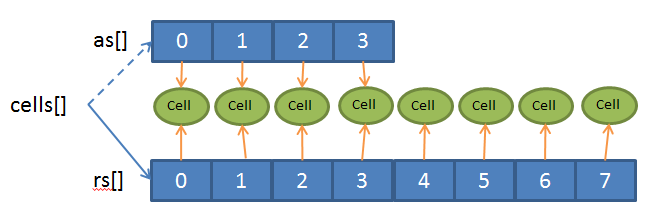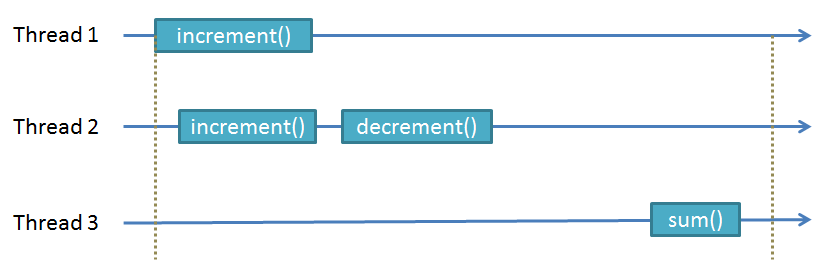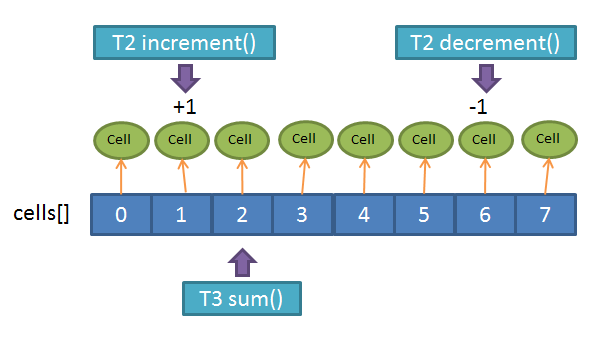## Thursday, September 12, 2013

### LongAdder is not Sequentially Consistent

Still on the topic of Counters, in this post we're going to talk a bit about LongAdder.
LongAdder is part of JDK 8, was done by Doug Lea, and there is a funny post about it on this blog that covers a good deal of its capabilities:
http://psy-lob-saw.blogspot.co.uk/2013/06/java-concurrent-counters-by-numbers.html
LongAdder is a scalable counter, and by "scalable" it means that it can scale almost linearly with the number of threads incrementing/decrementing the counter.

As the title of this post indicates, LongAdder is not Sequentially Consistent (SC)... well that isn't completely true because (as far as we could see) it is SC if we stick to using only increment() and sum() as will be shown below.
If you don't know what Sequential Consistency is, then most likely this doesn't affect you in any way, but if you want to use LongAdder as part of a finely synchronized mechanism, then you may start seeing strange effects.
http://en.wikipedia.org/wiki/Consistency_model

We're only interested in some of the details of the mechanism, so lets dive right in.
LongAdder.add(), which is called by increment() and decrement(), can sometimes decide to enlarge the array of Cells by calling longAccumulate()

public void add(long x) {
Cell[] as; long b, v; int m; Cell a;
if ((as = cells) != null || !casBase(b = base, b + x)) {
boolean uncontended = true;
if (as == null || (m = as.length - 1) < 0 ||
(a = as[getProbe() & m]) == null ||
!(uncontended = a.cas(v = a.value, v + x)))
longAccumulate(x, null, uncontended);
}
}

and to do so, it creates a new array temporarily in rs[] with double the size of the original array. If everything goes according to planed then cells[] will be pointing to the new array, where each entry of the array will be pointing to an Cell instance (or null):The code for longAccumulate() is long and complex so I'm not going to go over the details.
The interesting part is that when the sum() function is called, it may be running on a different array than an increment() running in another thread, because the sum() might have read the value of cells[] before it swapped to the new one, while the increment() saw the most recent one.

Even more interesting, is the fact that the same thread doing two consecutive add() will not necessarily write in the same cell a because the variable m might have changed or getProbe() may return a different result, and this is the one responsible for the usage of increment()/decrement() not being sequentially consistent.
The easiest is to give an example. Suppose we start with the counter at zero, and have three threads T1, T2 and T3, where T1 is doing an add() that will trigger an enlargement of the cells[] array, while T2 is doing an increment() followed by a decrement(), and T3 is doing a sum() call:By the sequential consistency rules, the fact that T2 does an increment() followed by a decrement() imposes this ordering on other threads, meaning that another thread may "see" just the increment(), or "see" the increment() and then the decrement(), but never the decrement() and then the increment(). In other words, when T3 calls sum() it may see 0 or 1, but it can never see -1.
Unfortunately, for the LongAdder, it can happen that it sees -1 (a very rare event, but possible). Here's how it happens:T3 may start the sum() to add up all the values of each cell in the cells[] array, an by the time it goes half-way through, T2 will call increment() which will increase cells by 1. Immediately afterwards (maybe T3 is sleeping) T2 will call decrement() but by this time T1 has changed the size of the array or the value returned by getProbe() and the entry allocated to T2 is now cells which it decrements to -1. T3 will then continue and see the value of -1 in cells, returning a sum value of -1, which is not sequentially consistent.

The logic for why the reset() is also not SC is similar to this same mechanism, so we will fore-go the example and description.

It seems like the only way to be SC is if only the sum() and increment() functions are used, because in that case, the counter is increasing monotonically, which means there is no way to discern which increment() was before or after, because they "are all the same".
If add() with different values are used, then the logic is the same as for the increment()/decrement(), and in that case it is not SC.

Stay tuned for the next post and you'll find out why   ;)

1.2.